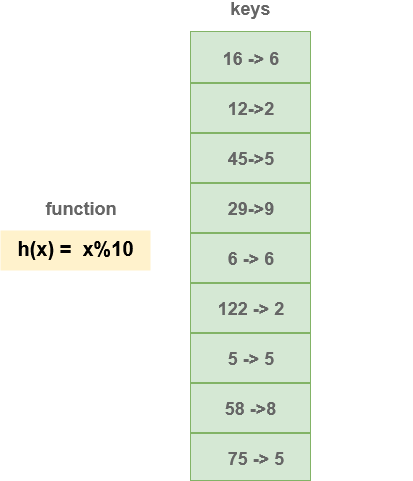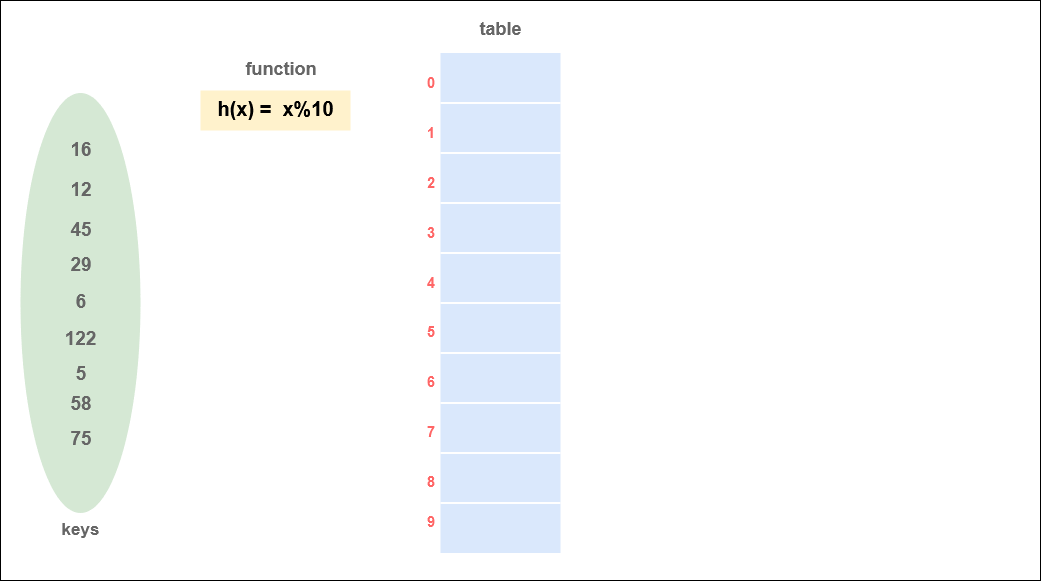# Chaining

Chaining is a collision resolution technique used when a hash function provides the same index for multiple values. These elements are stored at the same index by making use of a linked list data structure.
For example, if the hash function being used is `h(k) = k % 10` for the keys : `{13, 33, 67, 87}`
then,

``````h(13) = h(33) = 3
h(67) = h(87) = 7  ``````

The hash function is generating the same index for two different values. Both the values can’t be stored in the same place in the hash table.
Therefore we need to make use of a linked list to store the values. The hash table is made of array of linked lists here.

## Insertion

• If N is the number of possible key combinations generated by the hash function, then make a hash table of size `N.`
• Every index in the hash table will correspond to a linked list i.e., the hash table will contain a pointer to the head of the linked list of elements.
• Each slot for multiple elements contains a pointer to the head of the linked list. If there is no element present, the slot contains null.
• If some key comes into the hash table and the head is null, then that value is directly inserted in the head node.
• If a location in the hash table contains data, a new node will be created and added next to the head node.

Suppose we have data: `{16, 12, 45, 29, 6, 122, 5, 58, 75}` and the hash function is `h(x) = x%10`,
Keys will be :Hash Function: `h(x) = x%10`

``````int hash(int key)
{
return key%10;
}``````

Insertion in a Hash Table using chaining :#### Code for Insertion in Hash Table using Chaining :

Structure of Hash Table

``````struct Node *HT; // Hash Table will be an array of pointers
for(i=0;i<10;i++)
HT[i]=NULL;//initialise each slot with NULL pointers``````

Function for sorted Insert in a linked list :

``````void HashInsert(int val)
{
//create a newnode with val
struct node *newNode = malloc(sizeof(struct node));
newNode->data = val;
newNode->next = NULL;

//calculate hash index
int index = hash(val);

//check if HT[index] is empty
if(HT[index] == NULL)
HT[index] = newNode;
//collision
else
{
//add the node at the end of HT[index].
struct node *temp = HT[index];
while(temp->next)
{
temp = temp->next;
}

temp->next = newNode;
}
}``````

## Deletion

Deletion in the chained hash table is similar to that of deletion of a node from the linked list.
After the chain found, we have to use linked list deletion algorithm to remove the element.

• Compute the key for the value `val` to be deleted
• Using linked list deletion operation, delete the element.
• If operation successful return 1 else 0

Hash Function: `h(x) = x%10`

``````int hash(int key)
{
return key%10;
}``````

Delete Function

``````// function returns 1 if operation is successful
int HashDelete(int val)
{
int index = hash(val);
struct node *temp = HT[index], *dealloc;
if(temp != NULL)
{
if(temp->data == val)
{
dealloc = temp;
temp = temp->next;
free(dealloc);
return 1;
}
else
{
while(temp->next)
{
if(temp->next->data == val)
{
dealloc = temp->next;
temp->next = temp->next->next;
free(dealloc);
return 1;
}
temp = temp->next;
}
}
}

return 0;
}``````

## Searching

• Compute the hash key for the value asked
• Search the value in the entire linked list.
• Return 1 if found else 0
`````` int Search(int val)
{
int index = hash(val);
struct node *temp = HT[index];
while(temp)
{
if(temp->data == val)
return 1;
temp = temp->next;
}
return 0;
}``````

### Time Complexity

Analysis of Hash tablel is performed on basis of Loading Factor and not the number of keys.
If n is the Number of keys to be inserted in hash table,